﻿ 存在投机商和策略型消费者的零售商定价研究文章快速检索 高级检索

1. 湖北物流发展研究中心, 武汉 430205;
2. 西南交通大学 经济管理学院, 成都 610031

Optimal pricing research with speculators and strategic consumers
GUAN Zhen-zhong1,2, LI Wei2
1. Research Center of Hubei Logistics Development, Wuhan 430205, China;
2. School of Economics and Management, Southwest Jiaotong University, Chengdu 610031, China
Abstract:With the rapid changes in demand, lots of hot-selling products are subject to inventory and production constraints in the short term, resulting in the emergence of speculators. In addition, strategic consumer's delay-buying behavior will do a significant impact on the retailer's pricing decisions and revenue. In this paper, we consider a model that the monopoly retailer with fixed inventory makes pricing decisions when facing speculators. We use the Stackelberg model to analyze the speculators and strategic consumers' behavior, then we discuss the optimal price and retailer's revenue with strategic consumers proportions, consumer's reservation price variation coefficient, purchasing probability in period 2, and get the results of retailer pricing decisions. The conclusions show that the existence of speculators is benefit for retailers under specific situations.
Key words: speculators     strategic consumers     pricing     retailer

0 引言

4) 消费者保留价格会随着销售期的变化而改变,本文假设变动系数为$\alpha$,其中$\alpha\geq 1$.

5) 周期1进入市场并且购买产品的投机商的数量为$S$.

6) 将消费者分为两种类型,第一种称为固定消费者(fixed consumer),其数量为$W$; 第二种称为随机消费者(random consumer),其数量为$X$.

7) 固定消费者中,策略型消费者所占比例为$$(0\leq \leq1),那么短视型消费者所占比例为(1-). 8) 策略型消费者在周期2购买到产品的概率为\beta,其中0\leq\beta\leq 1. 9) 产品的单位成本、周期2结束后未卖出的产品残值均为0. 本文模型描述如下: 周期1,零售商首先制定一个产品价格决策p_1. 固定消费者W进入市场,其中短视型消费者根据自身保留价格的大小以及产品价格p_1决定购买或者离开市场; 策略型消费者根据自身保留价格、周期2的期望价格和周期2获得产品的概率,决定是立即购买还是延迟到周期2购买; 投机商根据周期2能够获得的期望利润决定是否购买产品. 周期2,零售商和投机商共同在市场中进行产品买卖行为. 而周期2新进入的消费者X和周期1选择推迟购买的策略型消费者一起共同组成产品购买者. 投机商根据零售商在周期2制定的价格p_2和X数量的大小决定产品的价格. 整个决策过程如图 1所示.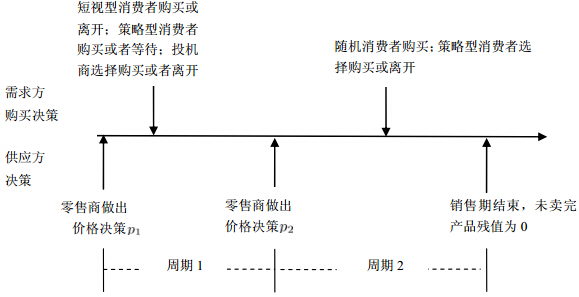图 1 零售商、投机商和消费者决策示意图 2 建立模型 2. 1 周期1 零售商在周期1的产品数量为K,设定的价格为p_1. 数量为W的固定消费者进入市场后,采取购买行动的短视型消费者的数量为(1-)W\frac{V_{H}-p_1}{V_{ H}-V_{ L}},其余的短视型消费者将退出市场. 当且仅当V-p_1\geq \beta(\alpha V-p_2)时,策略型消费者不会等待而立即购买该产品,可以得到消费者的保留价格为:  V=\frac{p_1-\beta p_2}{1-\alpha\beta} (1) 那么,周期1就采取购买行为的策略型消费者的数量为W\frac{V_{ H}-V}{V_{ H}-V_{ L}}. 另外,周期1购买产品的投机商的数量为S. 综上所述,周期1的产品销量为(1-)W\frac{V_{ H}-p_1}{V_{H}-V_{ L}}+ W\frac{V_{ H}-V}{V_{ H}-V_{ L}}+S,记为K_1. 因此,零售商周期1的收益为:  \pi _{1R} = \left\{ {\frac{W}{V_{ H} - V_{ L} }(V_{ H} - ( {1 - } )p_1 - V) + S} \right\}p_1 (2) 2. 2 现场销售期 周期2,零售商拥有的库存数量为K-K_1,投机商拥有的库存数量为S. 因此,此时供应方的产品库存数量为S+K-K_1. 消费者的数量包括选择等待在周期2购买的策略型消费者数量W_2=W\frac{V-p_1}{V_{ H}-V_{L}}和周期2新进入的随机消费者数量X,即消费者的数量总数为X+W_2. 根据周期2进入市场的随机消费者数量,本文将分三种情况讨论:随机消费者X很小,以至于W_2+X<S的情况; 随机消费者X中等,即S\leq W_2+X① W_2+X<S时,即周期2实际市场需求量很小. 投机商会将产品价格设定为消费者最低保留价格V_{ L},以便将库存中的产品全部卖出,此时零售商的销售量为0. 因此,零售商两周期的总收益为周期1的收益:  \pi = \pi _{1R} = \left\{ {\frac{W}{V_{ H} - V_{ L} }(V_{ H} - \left( {1 - } \right)p_1 - V) + S} \right\}p_1 (3) 令\frac{\partial \pi }{\partial p_1 } = 0,得到周期1的价格:  p_1 = \frac{\left( {1 - \alpha \beta } \right)(\left( {V_{ H} - V_{ L} } \right)S + WV_{ H} ) + \beta Wp_2 }{2W\left( {\alpha \beta - \alpha \beta + 1} \right)} (4) 由于零售商在周期2不能卖出任何产品. 因此,他们可以将产品的价格设定为任何值,不失一般性,这里将产品的价格设定为p_2=V_{ L}. 此时,零售商的收益为:  \pi ^\ast = \frac{(\left( {1 - \alpha \beta } \right)(V_{ H} W + \left( {V_{ H} - V_{ L} } \right)S) + \beta WV_{ L} )^2}{4W\left( {V_{ H} - V_{ L} } \right)\left( {1 - \alpha \beta } \right)\left( {\alpha \beta - \alpha \beta + 1} \right)} (5) 故可以得到以下结论: 定理1 给定消费者保留价格区间、投机商和固定消费者的数量,则可以得到零售商的最优价格策略p_1^{*}(\alpha,\beta)和p_2^*,以及零售商的最大收益\pi^*(\alpha,\beta). ② 当S\leq W_2+X因此,零售商周期2的利润为:  \pi _{2R} = p_2 \left( {X + W_2 - S} \right) (6) 其中,W_2 = W\frac{V - p_1 }{V_{ H} - V_{ L} }. 可以得到\pi _{2R} = p_2 ( {X + W\frac{V - p_1 }{V_{ H}- V_{ L} } - S} ). 将公式(1)代入公式(6),并令\frac{\partial \pi_{2R}}{\partial p_2}=0,可以得到零售商周期2制定的价格:  p_2 = \frac{\alpha p_1 }{2} + \frac{\left( {1 - \alpha \beta } \right)\left( {X - S} \right)\left( {V_{ H} - V_{ L} } \right)}{2\beta W} (7) 零售商两周期的总收益为:  \pi = \pi _{1R} + \pi _{2R} = p_2 \left( {X + W_2 - S} \right) + \left\{ {\frac{W}{V_{ H} - V_{ L} }(V_{ H} - \left( {1 - } \right)p_1 - V) + S} \right\}p_1 (8) 将所得价格p_2 和公式(1)代入(8)式中,并令\frac{\partial\pi}{\partial p_1}=0,可以得到周期1的最优价格:  p_1 = \frac{\left( {1 - \alpha \beta } \right)\left( {V_{ H} - V_{ L} } \right)((S\left( {1 - \alpha } \right) + X\left( {1 + \alpha } \right)) + 2V_{ H} W)}{W\left( {4 - 4\alpha \beta + 2\alpha \beta - \alpha ^2\beta } \right)} (9) 由此,可以得到如下命题. 命题 在上述两周期的零售商动态定价中, 如果已知策略型消费者所占比例$$ $(0\leq\leq 1)$,消费者周期2获得产品的风险系数$\beta$ $(0\leq\beta\leq 1)$,则可以得到零售商的最优价格策略:
 $p_1^\ast = \frac{\left( {1 - \alpha \beta } \right)\left\{ {\left( {V_{ H} - V_{ L} } \right)\left[{S\left( {1 - \alpha } \right) + X\left( {1 + \alpha } \right)} \right] + 2V_{ H} W} \right\}}{W\left( {4 - 4\alpha \beta + 2\alpha \beta - \alpha ^2\beta } \right)}$ (10)
 $p_2^\ast = \frac{\alpha p_1^\ast }{2} + \frac{\left( {1 - \alpha \beta } \right)\left( {X - S} \right)\left( {V_{ H} - V_{ L} } \right)}{2\beta W}$ (11)
 $V^\ast = \frac{p_1^\ast - \beta p_2^\ast }{1 - \alpha \beta }$ (12)
 $\pi ^\ast = \left( {X + W_2 - S} \right)p_2 ^\ast + \left\{ {\frac{W}{V_{ H} - V_{ L} }\left[{V_{ H} - \left( {1 - } \right)p_1 ^\ast - V^\ast } \right] + S} \right\}p_1 ^\ast$ (13)

③当$W_2+X\geq K_2+S$时,即周期2实际市场需求量很大. 此时,投机商会将产品的价格设定为最高保留价格$V_{ H}$,以便获取最大的利润. 由于消费者的数量足够大,零售商不用担心周期2产品卖不出去,就不会对产品打折,即周期2价格决策为$p_2=p_1$. 此时,消费者会首先从零售商那里购买产品,未在零售商那里购买到产品的消费者会转向投机商购买,但零售商和投机商的库存都会销售完. 此时,零售商卖出产品的数量为$K-K_1$,零售商周期2的收益为:
 $\pi _{2R} = \left( {K - K_1 } \right)p_2 = \left( {K - K_1 } \right)p_1$ (14)

 $\pi = \pi _{1R} + \pi _{2R} = Kp_1$ (15)
3 数值分析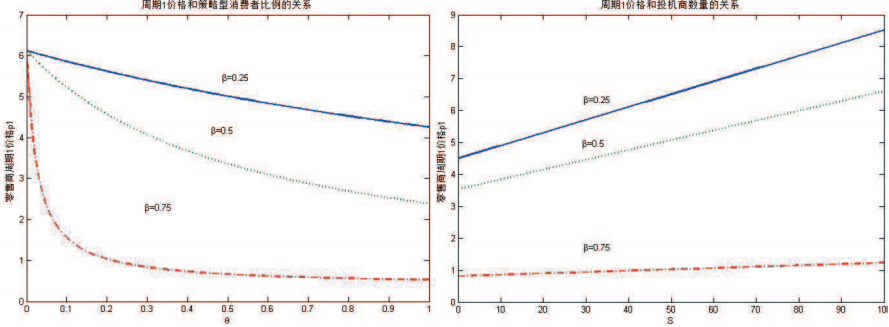图 2 零售商周期1产品价格和策略型消费者、投机商数量的关系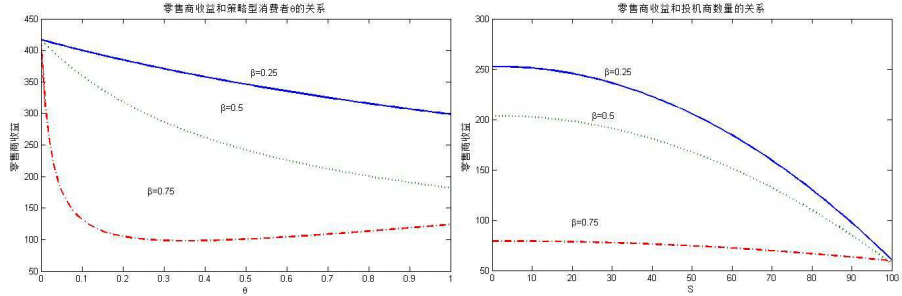图 3 零售商收益和策略型消费者、投机商数量的关系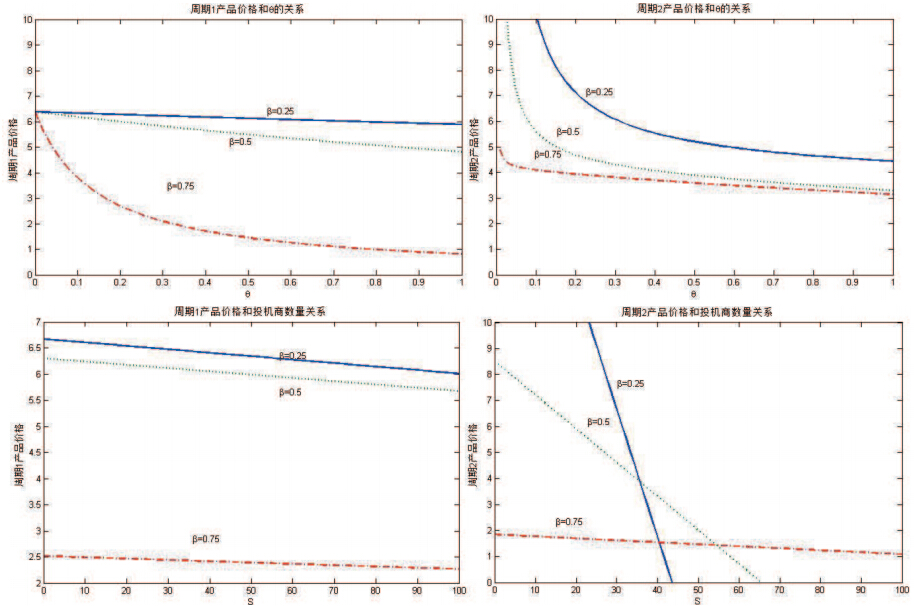图 4 零售商两周期产品价格和投机商数量的关系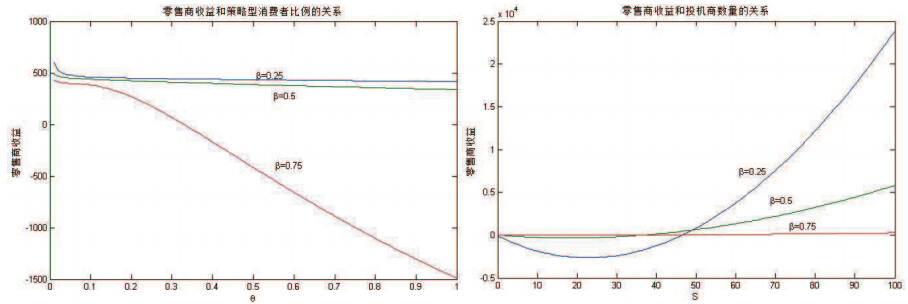图 5 零售商收益和策略型消费者、投机商数量的关系

  Mulpuru S, Hult P. Myths and truths about online customer reviews[R]. New York, Forrester Research, 2008.  Shen Z J, Su X M. Customer behavior modeling in revenue management and auctions: A review and new research opportunities[J]. Production and Operations Management, 2007, 16(6): 713-728.  Harris M, Raviv A. A theory of monopoly pricing schemes with demand uncertainty[J]. American Economy Review, 1981, 71(3): 347-365.  Talluri K, van Ryzin G. The theory and practice of revenue management[M]. Springer, New York, 2005.  Ovchinnikov A, Milner J M. Strategic response to wait-or-buy: Revenue management through last minute deals in the presence of customer leaning[R]. Working Paper, University of Toronto, 2005.  Aviv Y, Pazgal A. Optimal pricing of seasonal products in the presence of forward-looking consumers[J]. Manufacturing & Service Operations Management, 2008, 10(3): 339-359.  Su X. Intertemporal pricing with strategic customer behavior[J]. Management Science, 2007, 53(5): 726-741.  Elmalghraby W, Gulcu A, Keskinocak P. Optimal markdown mechanisms in the presence of rational customers with multi-unit demands[J]. Manufacturing Service & Operations Management, 2008, 10(1): 126-148.  Levin Y, McGill J, Nediak M. Price guarantees in dynamic pricing and revenue management[J]. Operations Research, 2007, 55(1): 75-97.  Levin Y, McGill J, Nediak M. Optimal dynamic pricing of perishable items by a monopolist facing strategic consumers[J]. Production and Operations Management, 2010, 19(1): 40-60.  Levin Y, Mcgill J, Nediak M. Dynamic pricing in the presence of strategic consumers and oligopolistic competition[J]. Management Science, 2009, 55(1): 32-46.  Zhang D, Cooper W L. Managing clearance sales in the presence of strategic customers[J]. Production and Operations Management, 2008, 17(4): 416-431.  刘晓峰, 黄沛. 基于策略型消费者的最优动态定价与库存决策[J]. 管理科学学报, 2009, 12(5): 18-26.Liu Xiaofeng, Huang Pei. Optimal dynamic pricing and inventory policy under strategic customers[J]. Journal of Management Sciences in China, 2009, 12(5): 18-26.  杨慧, 周晶, 宋华明. 考虑消费者短视和策略行为的动态定价研究[J]. 管理工程学报, 2010, 24(4): 133-137.Yang Hui, Zhou Jing, Song Huaming. A dynamic pricing model with strategic and myopic consumers[J]. Journal of Industrial Engineering/Engineering Management, 2010, 24(4): 133-137.  Swinney R. Selling to strategic consumers when product value is uncertain: The value of matching supply and demand[J]. Management Science, 2011(6): 1-15.  Swofford J. Arbitrage speculation and public policy toward ticket scalping[J]. Public Finance Review, 1999, 27(5): 531-540.  Courty P. Some economics of ticket resale[J]. Economy Perceptions, 2003, 17(2): 85-97.  Karp L, Perloff J. When promoters like scalpers[J]. Economy Management Strategy, 2005, 14(2): 477-508.  Su X. Optimal pricing with speculators and strategic consumers[J]. Management Science, 2010, 56(1): 25-40.  Wei S L, Tang C S. Advance selling in the presence of speculators and forward-looking consumers[J]. Production and Operations Management, 2013, 22(3): 571-587.
0

#### 文章信息

GUAN Zhen-zhong, LI Wei

Optimal pricing research with speculators and strategic consumers

Systems Engineering - Theory & practice, 2015, 35(2): 308-314.